# 六、构造和建立直方图

• 直方图的理论介绍
• 灰度直方图
• 颜色直方图
• 直方图的自定义可视化
• 比较 OpenCV，NumPy 和 Matplotlib 直方图
• 直方图均衡
• 自适应直方图均衡
• 比较 CLAHE 和直方图均衡
• 直方图比较

# 技术要求

• Python 和 OpenCV
• 特定于 Python 的 IDE
• NumPy 和 Matplotlib 包
• Git 客户端

# 直方图的理论介绍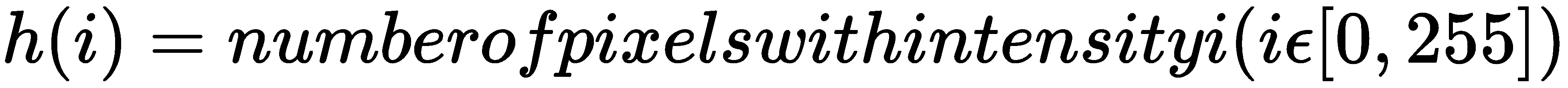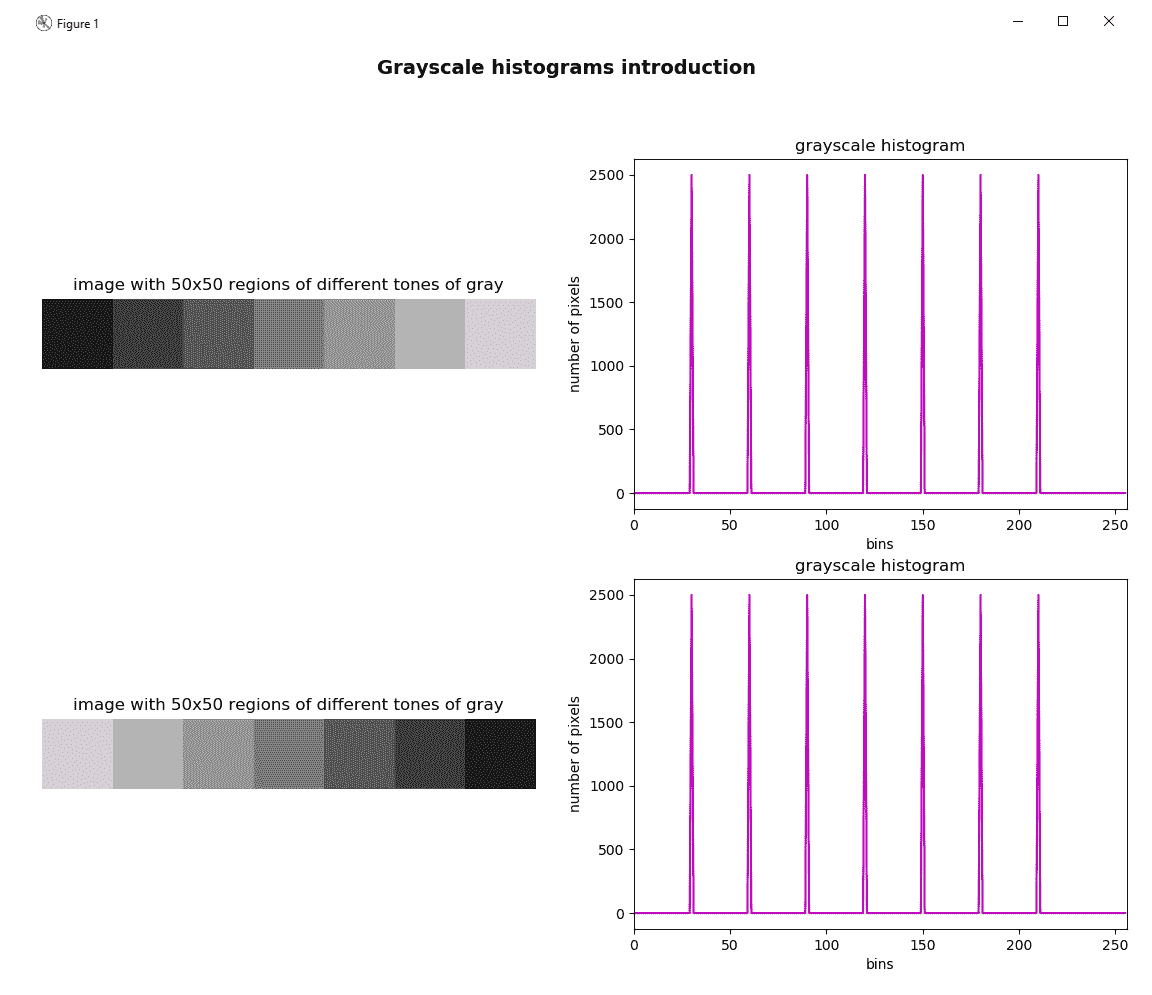histogram_introduction.py脚本如前所示绘制图形。 在此脚本中，build_sample_image()函数使用 NumPy 操作构建第一张图像（上），build_sample_image_2()函数构建第二张图像（下）。 接下来提供build_sample_image()的代码：

def build_sample_image():
"""Builds a sample image with 50x50 regions of different tones of gray"""

# Define the different tones. In this case: 60, 90, 120, ..., 210
# The end of interval (240) is not included
tones = np.arange(start=60, stop=240, step=30)

# Initialize result withe the first 50x50 region with 30-intensity level
result = np.ones((50, 50, 3), dtype="uint8") * 30

# Build the image concatenating horizontally the regions:
for tone in tones:
img = np.ones((50, 50, 3), dtype="uint8") * tone
result = np.concatenate((result, img), axis=1)

return result



def build_sample_image_2():
"""Builds a sample image with 50x50 regions of different tones of gray
flipping the output of build_sample_image()
"""

# Flip the image in the left/right direction:
img = np.fliplr(build_sample_image())
return img


• np.ones()：返回给定形状和类型的数组，并填充1的值。 在这种情况下，形状为(50, 50, 3)dtype="uint8"
• np.arange()：考虑到提供的步骤，返回给定间隔内的均匀间隔的值。 不包括间隔的结尾（在这种情况下为240）。
• np.concatenate()：沿着现有轴连接一系列数组； 在这种情况下，axis=1可以水平连接图像。
• np.fliplr()：沿左右方向翻转数组。

# 直方图术语

• bins：上一个屏幕截图中的直方图显示了每个色调值的像素数（频率），范围从0255。 这些256值的每个在直方图术语中称为箱子。 可以根据需要选择bins的数量。 常用值为8163264128256。 OpenCV 使用histSize来引用bins
• range：这是我们要测量的强度值的范围。 通常，它是[0,255]，对应于所有色调值（0对应于黑色，255对应于白色）。

# 灰度直方图

OpenCV 提供cv2.calcHist()函数以便计算一个或多个数组的直方图。 因此，该函数可以应用于单通道图像（例如灰度图像）和多通道图像（例如 BGR 图像）。

cv2.calcHist(images, channels, mask, histSize, ranges[, hist[, accumulate]])


• images：它表示作为列表提供的uint8float32类型的源图像（例如[gray_img]）。
• channels：它代表我们计算其列表的直方图的通道索引（例如，对于灰度图像，；对于多通道图像， 分别计算第一，第二或第三通道的直方图）。
• mask：它代表一个遮罩图像，用于计算由遮罩定义的图像特定区域的直方图。 如果此参数等于None，则将在没有遮罩的情况下计算直方图，并且将使用完整图像。
• histSize：表示作为列表提供的bins的数量（例如）。
• ranges：它表示我们要测量的强度值的范围（例如[0,256]）。

# 没有遮罩的灰度直方图

image = cv2.imread('lenna.png')
gray_image = cv2.cvtColor(image, cv2.COLOR_BGR2GRAY)
hist = cv2.calcHist([gray_image], , None, , [0, 256])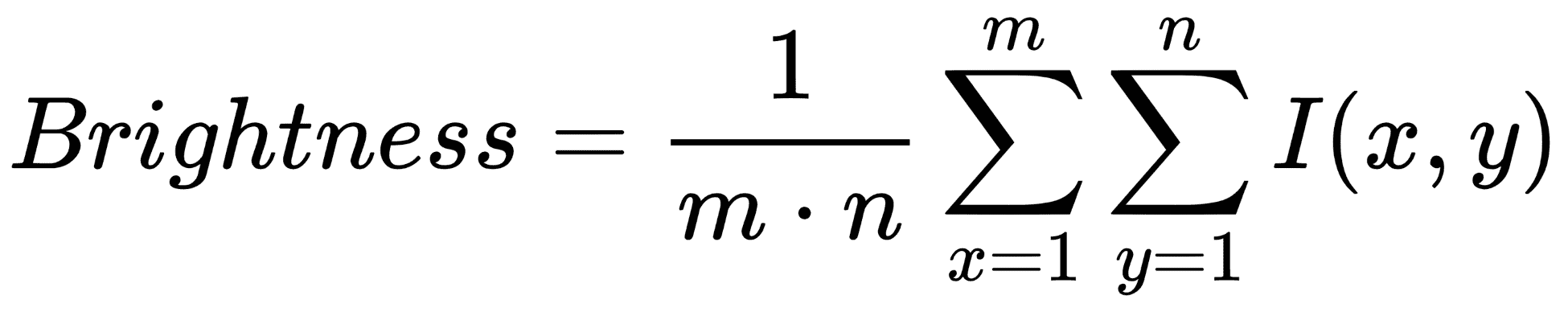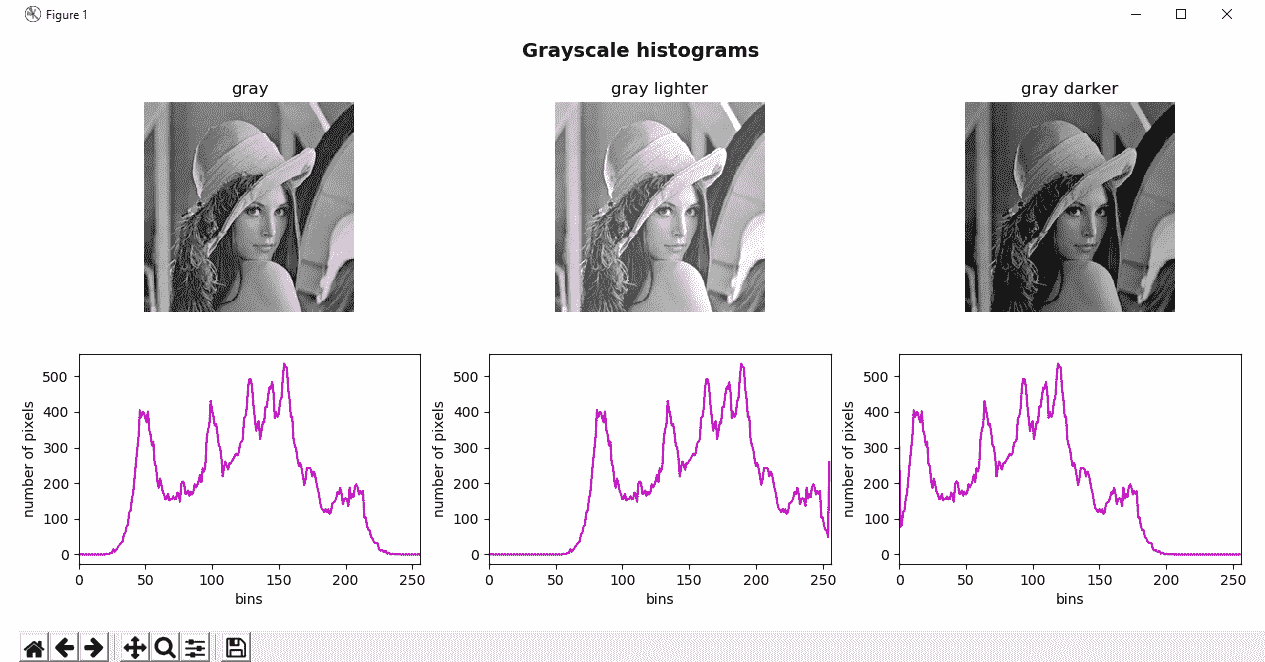# Add 35 to every pixel on the grayscale image (the result will look lighter) and calculate histogram
M = np.ones(gray_image.shape, dtype="uint8") * 35

# Subtract 35 from every pixel (the result will look darker) and calculate histogram
subtracted_image = cv2.subtract(gray_image, M)
hist_subtracted_image = cv2.calcHist([subtracted_image], , None, , [0, 256])


# 带遮罩的灰度直方图

mask = np.zeros(gray_image.shape[:2], np.uint8)


hist_mask = cv2.calcHist([gray_image], , mask, , [0, 256])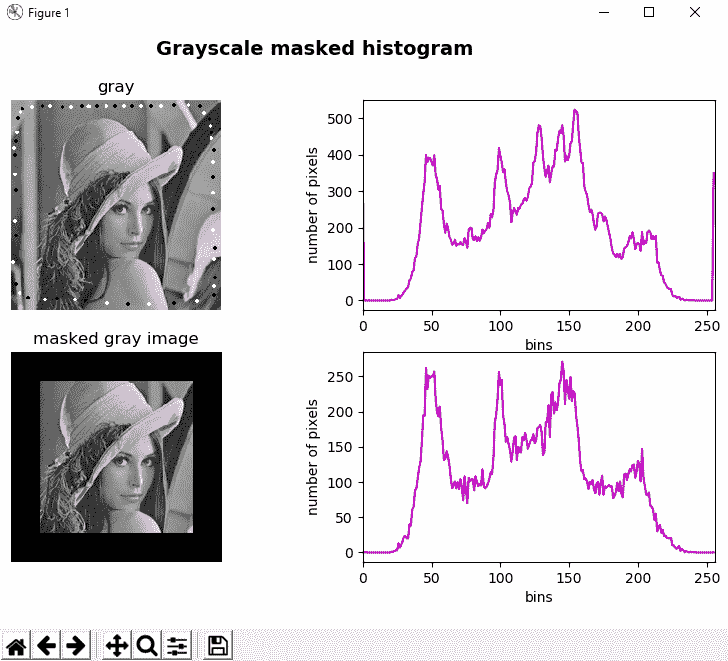# 颜色直方图

def hist_color_img(img):
"""Calculates the histogram from a three-channel image"""

histr = []
histr.append(cv2.calcHist([img], , None, , [0, 256]))
histr.append(cv2.calcHist([img], , None, , [0, 256]))
histr.append(cv2.calcHist([img], , None, , [0, 256]))
return histr


• 计算蓝色通道的直方图：cv2.calcHist([img], , None, , [0, 256])
• 计算绿色通道的直方图：cv2.calcHist([img], , None, , [0, 256])
• 计算红色通道的直方图：cv2.calcHist([img], , None, , [0, 256])

image = cv2.imread('lenna.png')
hist_color = hist_color_img(image)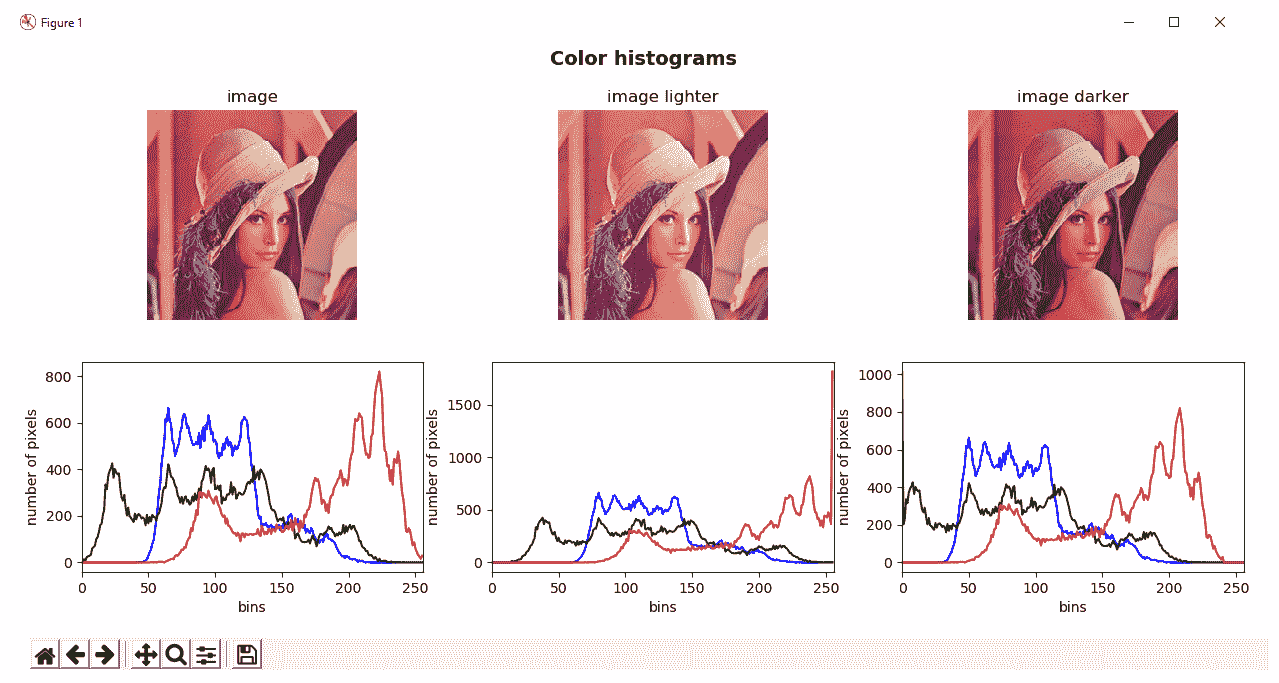# 直方图的自定义可视化

def plot_hist(hist_items, color):
"""Plots the histogram of a image"""

# For visualization purposes we add some offset:
offset_down = 10
offset_up = 10

# This will be used for creating the points to visualize (x-coordinates):
x_values = np.arange(256).reshape(256, 1)

canvas = np.ones((300, 256, 3), dtype="uint8") * 255
for hist_item, col in zip(hist_items, color):
# Normalize in the range for proper visualization:
cv2.normalize(hist_item, hist_item, 0 + offset_down, 300 - offset_up, cv2.NORM_MINMAX)
# Round the normalized values of the histogram:
around = np.around(hist_item)
# Cast the values to int:
hist = np.int32(around)
# Create the points using the histogram and the x-coordinates:
pts = np.column_stack((x_values, hist))
# Draw the points:
cv2.polylines(canvas, [pts], False, col, 2)
# Draw a rectangle:
cv2.rectangle(canvas, (0, 0), (255, 298), (0, 0, 0), 1)

# Flip the image in the up/down direction:
res = np.flipud(canvas)

return res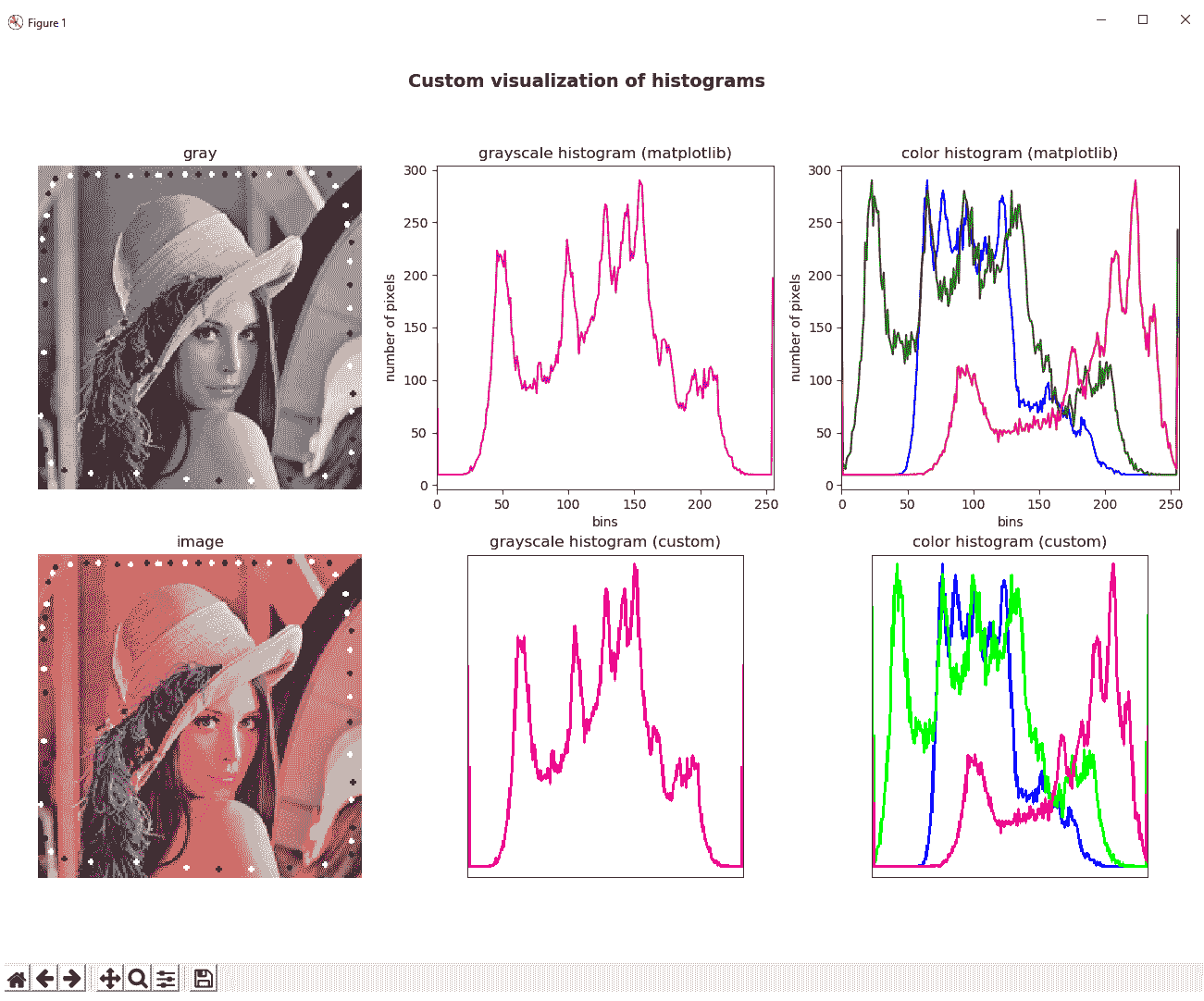# 比较 OpenCV，NumPy 和 Matplotlib 直方图

from timeit import default_timer as timer


start = timer()
# ...
end = timer()
execution_time = start - end


• cv2.calcHist()由 OpenCV 提供
• np.histogram()由 NumPy 提供
• Matplotlib 提供的plt.hist()

start = timer()
# Calculate the histogram calling cv2.calcHist()
hist = cv2.calcHist([gray_image], , None, , [0, 256])
end = timer()
exec_time_calc_hist = (end - start) * 1000

start = timer()
# Calculate the histogram calling np.histogram():
hist_np, bins_np = np.histogram(gray_image.ravel(), 256, [0, 256])
end = timer()
exec_time_np_hist = (end - start) * 1000

start = timer()
# Calculate the histogram calling plt.hist():
(n, bins, patches) = plt.hist(gray_image.ravel(), 256, [0, 256])
end = timer()
exec_time_plt_hist = (end - start) * 1000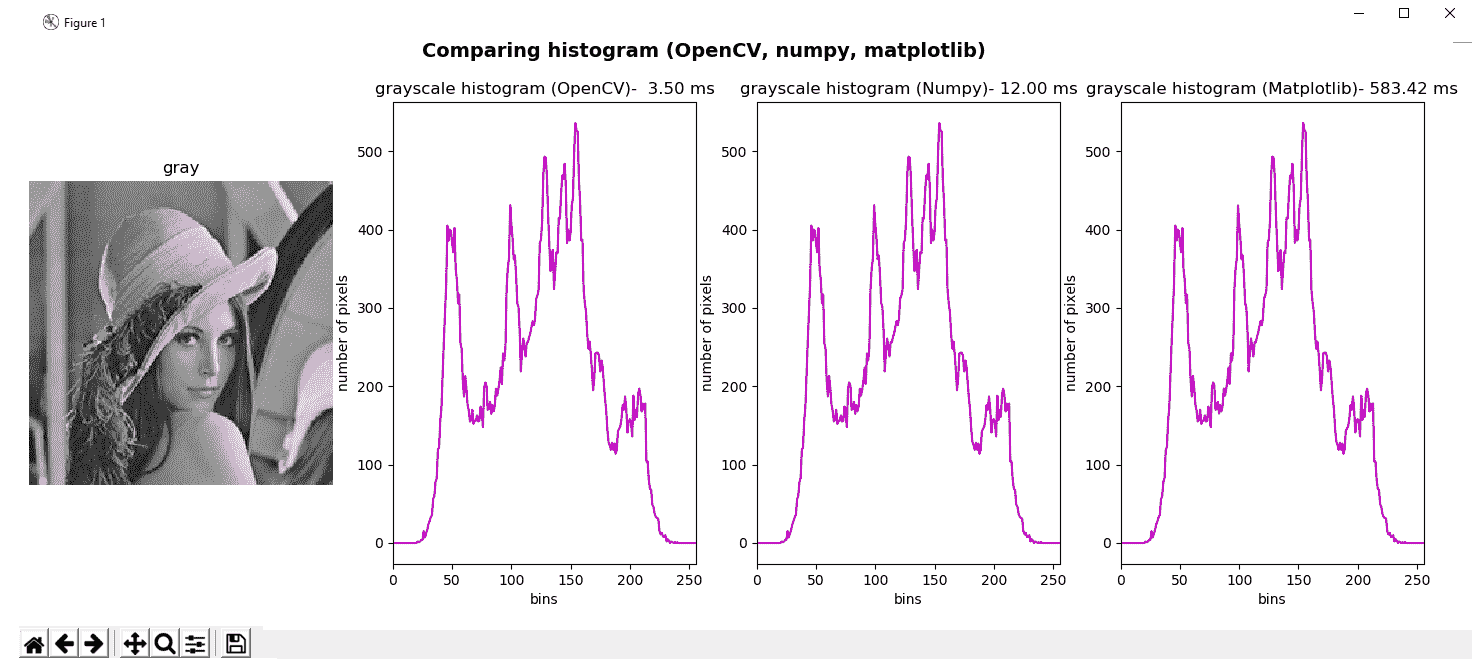# 灰度直方图均衡

image = cv2.imread('lenna.png')
gray_image = cv2.cvtColor(image, cv2.COLOR_BGR2GRAY)
gray_image_eq = cv2.equalizeHist(gray_image)


grayscale_histogram_equalization.py脚本中，我们将直方图均衡化应用于三个图像。 第一个是原始灰度图像。 第二个是原始图像，但是在我们已经向图像的每个像素添加35的意义上进行了修改。 第三个是原始图像，但是在我们已经从图像的每个像素中减去35的意义上进行了修改。 我们还计算了直方图均衡之前和之后的直方图。 最后，绘制所有这些图像。 在以下屏幕截图中可以看到此脚本的输出：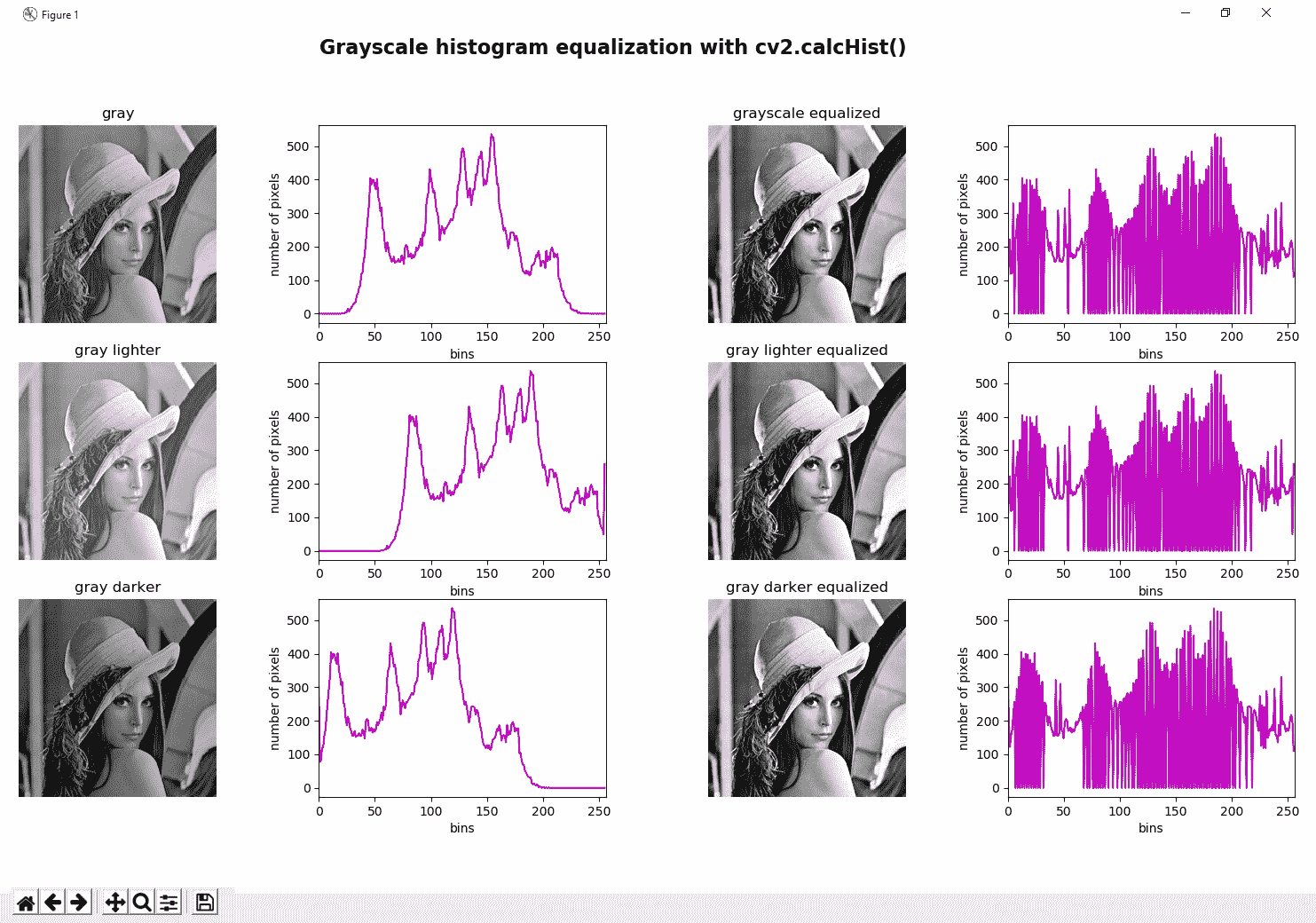# 颜色直方图均衡

def equalize_hist_color(img):
"""Equalize the image splitting the image applying cv2.equalizeHist() to each channel and merging the results"""

channels = cv2.split(img)
eq_channels = []
for ch in channels:
eq_channels.append(cv2.equalizeHist(ch))

eq_image = cv2.merge(eq_channels)
return eq_image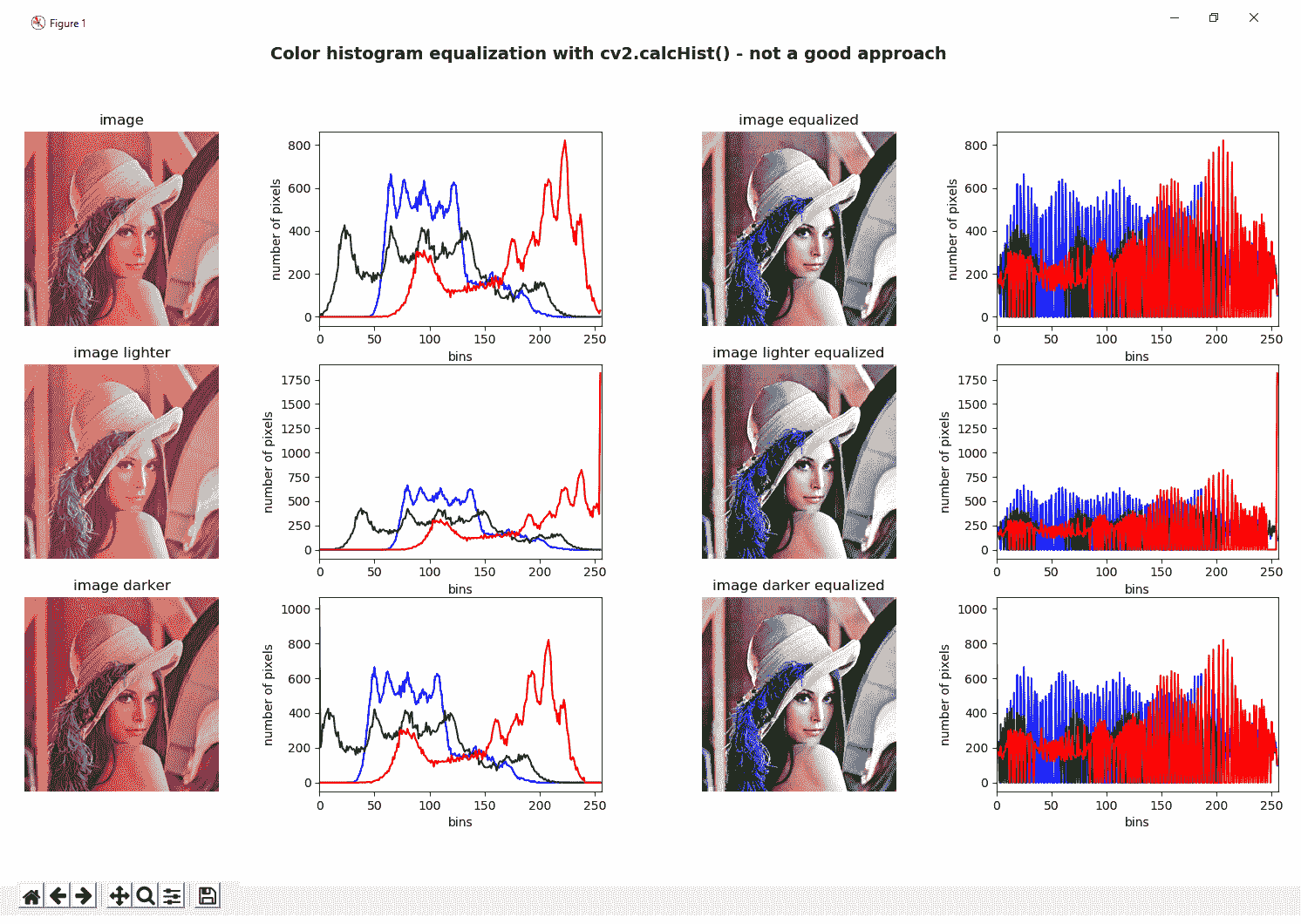color_histogram_equalization_hsv.py脚本中可以看到这种方法，其中equalize_hist_color_hsv()函数将执行以下功能：

def equalize_hist_color_hsv(img):
"""Equalize the image splitting the image after HSV conversion and applying cv2.equalizeHist()
to the V channel, merging the channels and convert back to the BGR color space
"""

H, S, V = cv2.split(cv2.cvtColor(img, cv2.COLOR_BGR2HSV))
eq_V = cv2.equalizeHist(V)
eq_image = cv2.cvtColor(cv2.merge([H, S, eq_V]), cv2.COLOR_HSV2BGR)
return eq_image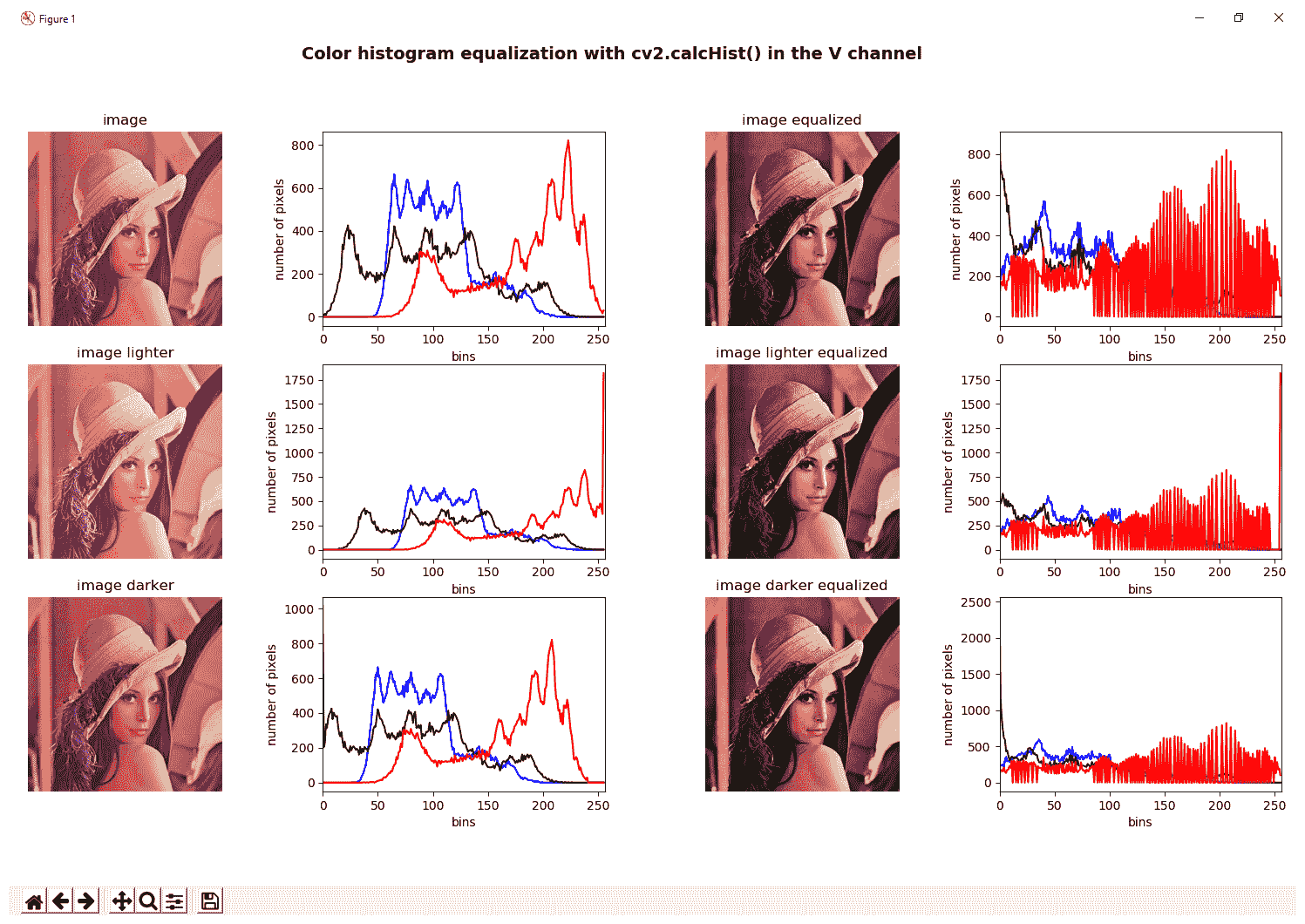# 对比度受限的自适应直方图均衡

clahe_histogram_equalization.py脚本中，我们将 CLAHE 应用于灰度和彩色图像。 应用 CLAHE 时，有两个参数需要调整。 第一个是clipLimit，它设置对比度限制的阈值。 默认值为40。 第二个是tileGridSize，它设置行和列中瓦片的数量。 当应用 CLAHE 时，图像被分成称为瓦片的小块（默认为8 x 8）以执行其计算。

clahe = cv2.createCLAHE(clipLimit=2.0)
gray_image_clahe = clahe.apply(gray_image)


def equalize_clahe_color_hsv(img):
"""Equalize the image splitting after conversion to HSV and applying CLAHE
to the V channel and merging the channels and convert back to BGR
"""

cla = cv2.createCLAHE(clipLimit=4.0)
H, S, V = cv2.split(cv2.cvtColor(img, cv2.COLOR_BGR2HSV))
eq_V = cla.apply(V)
eq_image = cv2.cvtColor(cv2.merge([H, S, eq_V]), cv2.COLOR_HSV2BGR)
return eq_image

def equalize_clahe_color_lab(img):
"""Equalize the image splitting after conversion to LAB and applying CLAHE
to the L channel and merging the channels and convert back to BGR
"""

cla = cv2.createCLAHE(clipLimit=4.0)
L, a, b = cv2.split(cv2.cvtColor(img, cv2.COLOR_BGR2Lab))
eq_L = cla.apply(L)
eq_image = cv2.cvtColor(cv2.merge([eq_L, a, b]), cv2.COLOR_Lab2BGR)
return eq_image

def equalize_clahe_color_yuv(img):
"""Equalize the image splitting after conversion to YUV and applying CLAHE
to the Y channel and merging the channels and convert back to BGR
"""

cla = cv2.createCLAHE(clipLimit=4.0)
Y, U, V = cv2.split(cv2.cvtColor(img, cv2.COLOR_BGR2YUV))
eq_Y = cla.apply(Y)
eq_image = cv2.cvtColor(cv2.merge([eq_Y, U, V]), cv2.COLOR_YUV2BGR)
return eq_image

def equalize_clahe_color(img):
"""Equalize the image splitting the image applying CLAHE to each channel and merging the results"""

cla = cv2.createCLAHE(clipLimit=4.0)
channels = cv2.split(img)
eq_channels = []
for ch in channels:
eq_channels.append(cla.apply(ch))

eq_image = cv2.merge(eq_channels)
return eq_image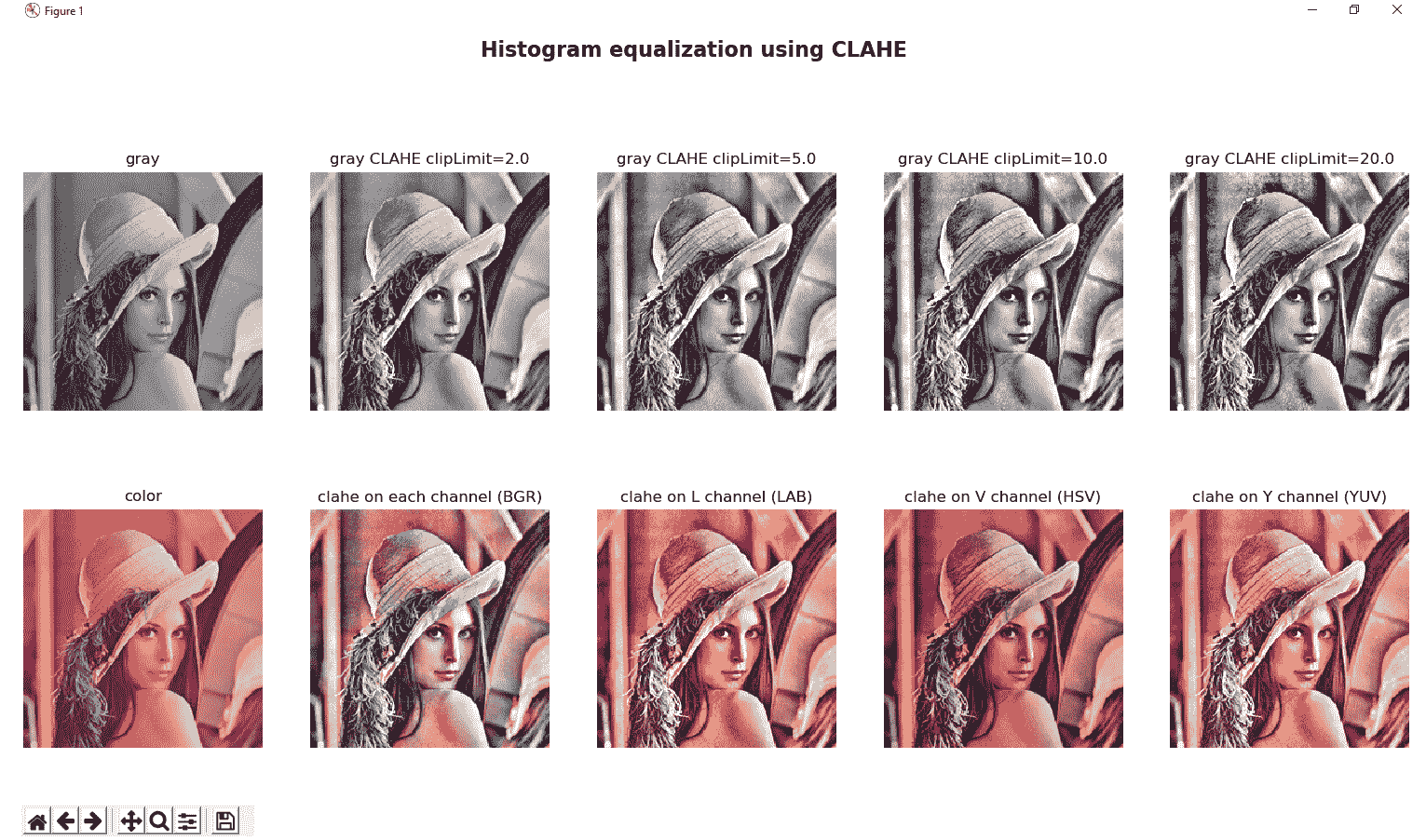# 比较 CLAHE 和直方图均衡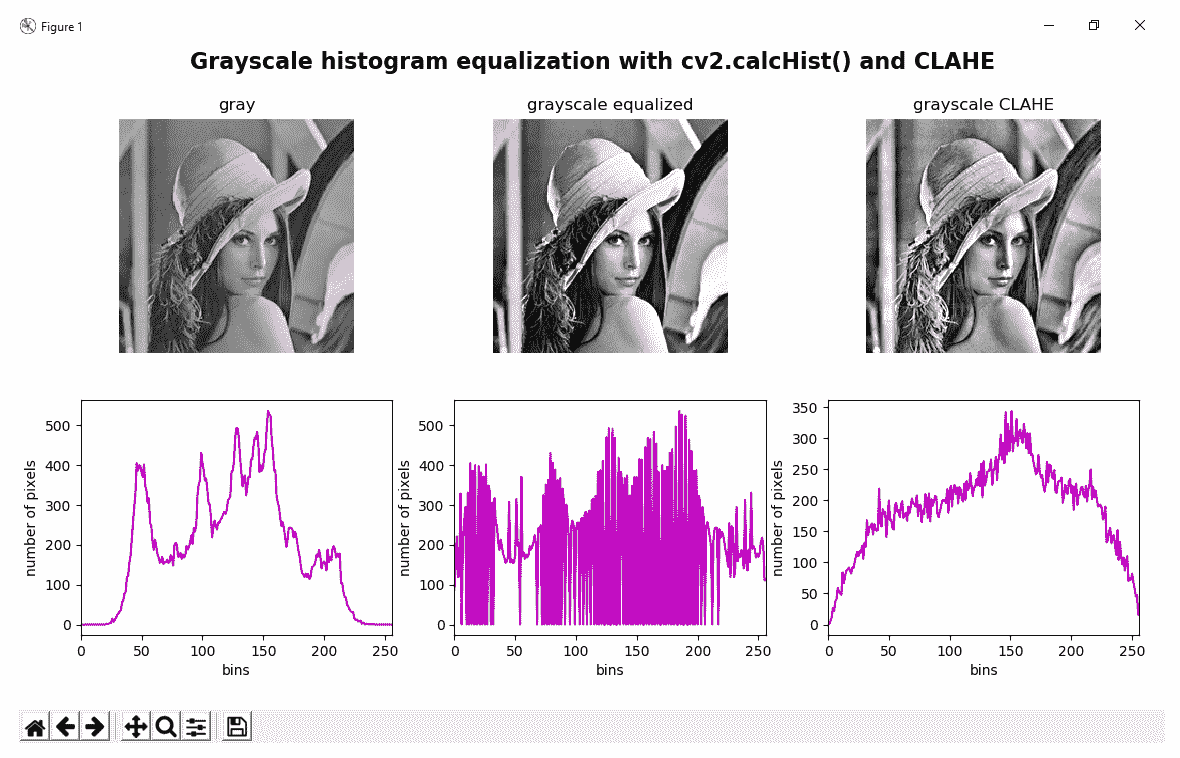# 直方图比较

OpenCV 提供的与直方图相关的一种有趣函数是cv2.compareHist()函数，该函数可用于获取一个数值参数，该数值参数表示两个直方图相互匹配的程度。 从这个意义上讲，由于直方图反映了图像中像素值的强度分布，因此该函数可用于比较图像。 如前所述，直方图仅显示统计信息，而不显示像素的位置。 因此，图像比较的常用方法是将图像划分为一定数量的区域（通常具有相同的大小），计算每个区域的直方图，最后将所有直方图连接起来以创建图像的特征表示 。 在此示例中，为简单起见，我们不会将图像划分为一定数量的区域，因此将仅使用一个区域（完整图像）。

cv2.compareHist()函数的签名如下：

cv2.compareHist(H1, H2, method)


OpenCV 提供了四种不同的度量标准（方法）来计算匹配项：

• cv2.HISTCMP_CORREL：此度量标准计算两个直方图之间的相关性。 此指标返回[-1, 1]范围内的值，其中1表示完美匹配，而-1完全不匹配。
• cv2.HISTCMP_CHISQR：此度量标准计算两个直方图之间的卡方距离。 此指标返回[0, unbounded]范围内的值，其中0表示完美匹配，而不匹配则不受限制。
• cv2.HISTCMP_INTERSECT：此度量标准计算两个直方图之间的交集。 如果直方图已归一化，则此度量标准将返回[0,1]范围内的值，其中1表示完美匹配，而0则完全不匹配。
• cv2.HISTCMP_BHATTACHARYYA：此度量标准计算两个直方图之间的 Bhattacharyya 距离。 此指标返回[0,1]范围内的值，其中0是完美匹配，而1完全不匹配。

compare_histograms.py脚本中，我们首先加载四个图像，然后使用先前注释的所有度量标准来计算所有这些图像与测试图像之间的相似度。

• gray_image.png：此图像对应于灰度图像。
• gray_added_image.png：此图像对应于原始图像，但在某种意义上进行了修改，即我们已经向图像的每个像素添加了35
• gray_subtracted_image.png：此图像对应于原始图像，但在某种意义上进行了修改，因为我们已经对图像的每个像素减去了35
• gray_blurred.png：此图像对应于原始图像，但已使用模糊过滤器（cv2.blur(gray_image, (10, 10)）进行了修改。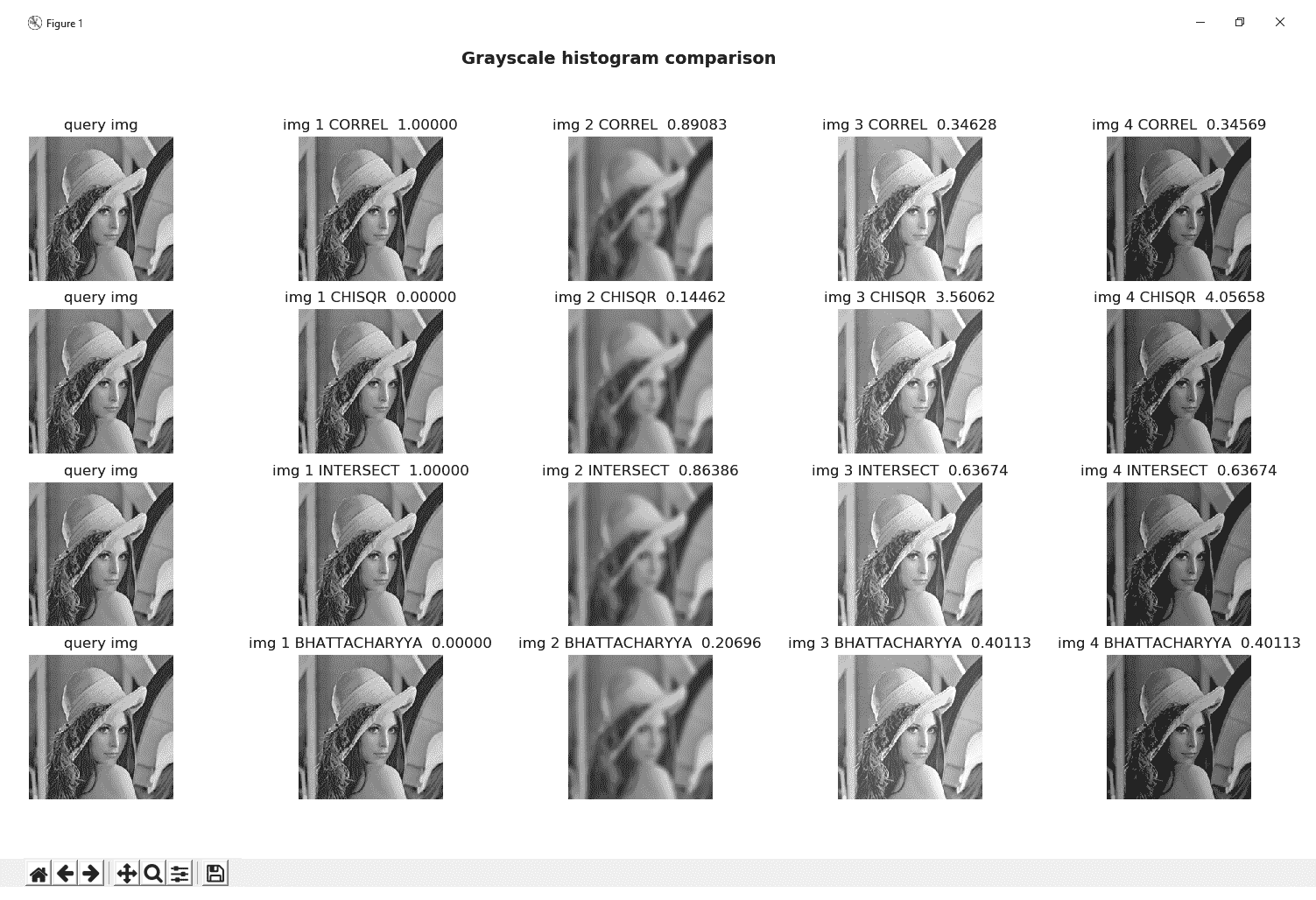# 问题

1. 什么是图像直方图？
2. 使用64箱计算灰度图像的直方图。
3. 50添加到灰度图像上的每个像素（结果看起来更亮）并计算直方图。
4. 计算没有遮罩的 BGR 图像的红色通道直方图。
5. OpenCV，NumPy 和 Matplotlib 提供哪些函数来计算直方图？
6. 修改grayscale_histogram.py脚本以计算这三个图像（gray_imageadded_imagesubtracted_image）的亮度。 将脚本重命名为grayscale_histogram_brightness.py
7. 修改comparing_hist_equalization_clahe.py脚本以显示cv2.equalizeHist()和 CLAHE 的执行时间。 将其重命名为comparing_hist_equalization_clahe_time.py